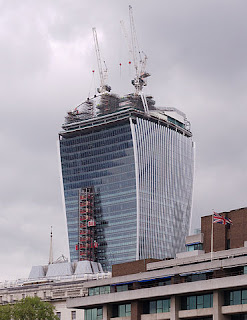## September 04, 2013

### The building that melted a Jaguar

Have you heard about the building in London that has been blamed for melting parts of a car by reflecting sunlight off of it?  It made the news today and was all over social media.Image Credit: Matt Buck
The first thing I noticed was that the building is curved both horizontally and vertically. I thought it would be interesting to try modeling the building as a spherical mirror and see what I could estimate from this model. Is the building a part of a sphere? Probably not.  Is it close enough to use as a model? That's the fun of trying to figure it out.

I found some renderings of the building on its website. I decided to use the 32nd floor to use to estimate the focal length of the horizontally curved part because it looked to be the most curved of all the floor plans in the linked PDF. Here's a screenshot from that file:

You can see the full size image if you click the image. What I liked about the rendering of the floor was that there were dimensions given for the usable office space on the floor. What I didn't like was that there didn't seem to be enough information to directly estimate the radius of a circle defined by the arc of the side of the building. (Maybe I'm wrong about that. It could be an interesting geometry problem. Paging Dan Meyer...)  I also didn't like the part about the floor plans not being to scale. I chose to ignore that part. I did like that units were in meters. Because physics.

My plan is to draw a circle so the side of the building follows the edge of the circle. Then, I'll use the dimension given in the image to figure out the size of circle.  From that I can get the focal length of a spherical mirror of that size.

Here's what the circle looks like when I put it up to the side of the building:

The blue bar is a rectangle the size of the arrow 20.5 m long in the center of the lower part of the drawing. The red section is the fraction of the circle that I could get into this image.  Here's the zoomed out view:

That's a big circle.  Let's figure out how big.  The blue box when I drew it (in Inkscape, if you're curious) was 431 units (whatever a unit is) long. So the scale is 431 units / 20.5 m or roughly 21.0 units / m.

The circle has a diameter of 10640 units.  So the physical diameter of the circle would be 10640 units / 21.0 units / m = 507 m.

The focal length of a spherical mirror is half the radius of the sphere. So the focal length I estimate from this floor is about 127 m.

Looking up the the height of the building gives a value of 180 m, according to Wikipedia and Google, the sources of all knowledge. Figuring out the height of the 32nd floor is not straightforward, but I estimated it to be about 142 meters.

So if the building could somehow reflect the sunlight straight down (which is can't, and doesn't) then according to my rough estimates, the focal length wouldn't be at ground level.  This makes me believe the following could all be true:
1. The light is reflected off of a lower floor.
2. The radius of curvature was underestimated.
3. The floor height was overestimated.
4. The ground level of the building is lower than where the car was sitting when it got melted.
5. I made some other miscalculation.
I'm actually satisfied that I'm on the same order of magnitude for the focal length and the height of the building.  If the lower floors (say the 16th) have a larger radius (less curved) and are obviously closer to the ground, then the focal length could easily be longer than the distance from the windows to the ground.

I think this is a neat way to apply some physics to an interesting news story. I have more to think about on this one. For now, I'll have to check and see what Rhett has to say about this building.